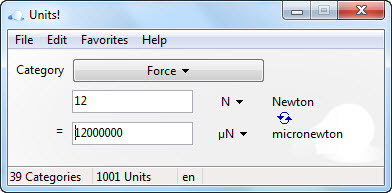# Free Unit and Currency Converter

Recently, I found a useful program online that can be use for the purpose of unit and currency conversion. This freeware program can assist in day to day unit conversion with over 1000 in its database. Students studying science subjects like chemistry, math, physics and any subjects that deal with Units will find this simple program very useful. The name of the freeware program that can be use for the sake of Unit & Currency Conversion is called UNIT!!UNIT can also be use for the conversion of currency of most commonly used international currencies. UNIT currency converter is always updated daily.

#### Below are the categories of what UNIT can convert to (more than 1000 units):

• Absorbed Energy
• Acceleration
• Amount of substance
• Angle
• Area
• Currencies
• Data Transfer Speed
• Data Volume
• Density
• Electric capacitance
• Electric charge
• Electric conductance
• Electric field
• Electrical impedance, resistance
• Electrical power
• Energy
• Force
• Frequency
• Inductance
• Length
• Luminous intensity
• Magnetic field
• Magnetic flux
• Magnetic flux density / induction
• Mass, Weight
• Moment of Inertia
• Power
• Pressure
• Roentgen Equivalent in Man
• Temperature
• Time
• Torque
• Velocity (angular)
• Velocity / Speed
• Velocity of Volume / Volume Speed
• Voltage / Potential differece
• Volume